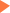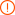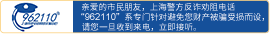# 新SAT官方Daily Practise每日一题数学函数题

2017年07月05日10:35 来源：小站教育作者：小站SAT编辑## SAT/ACT备考资料免费领取 请选择资料！

In triangle ABC, the sum of the measures of angle A and angle B is 70 degrees. The measure of angle A is one-half the measure of angle B. If the measure of angle B is equal to x degrees, which of the following expressions represents the sum of angles A,B and C?

A.x+2x+110

B.x/2+x+110

C.1/2(x+2x+110)

D.x/2+x+70

comma ['kɔmə] n. 逗号，停顿，间歇 n. 银纹多角蛱蝶

measure ['meʒə] n. 措施，办法，量度，尺寸 v. 测量，量

multiple ['mʌltipl] adj. 许多，多种多样 n. 倍数，并联

triangle ['traiæŋgl] n. 三角(形)

Choice B is correct. The sum of the three interior angles in a triangle is 180 degrees. If the measures of two interior angles in the triangle sum to 70 degrees, then the third angle must measure 110 degrees. If the measure of angle B is x and the measure of angle A is one-half of the measure of angle B comma then the measure of angle A is x/2. Therefore, x/2+x+110 is the sum of the measures of the angles in triangle ABC.

### 相关文章未输入验证码未输入验证码未输入验证码

## SAT30天热搜沪公网安备 31010602002658号